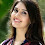## Tuesday, December 15, 2015

### Dimensional Formula and Unit of Work Done

Work can be represented in terms of dimensional formula and can be measured in different kinds of systems of measurement like SI system and CGS system.Work is said to be done when  applied force is able to produce displacement. Work is the dot product of force and displacement.

Dimensional formula is the representation of a physical quantity in terms of fundamental quantities. The powers to which fundamental quantities are raised to get the physical quantity are called dimensions. Dimensional formula is understanding a physical quantities and studying about what it consists of in terms of dimensional formula.

Work is the dot product of two vectors force and displacement. It means even when we multiply  two vectors, we are going to a scalar quantity.It means, we can understand the physical quantity in terms only magnitude and it does not need a direction to understand the work  done.

We can get the dimensional formula of work  done basing on the formula of work done and that is product of force and displacement in terms of magnitude.

We can also write it SI unit as joule and it can be even represented as J.  We can measure work done in CGS system with a unit called earg. These two are in the name of great scientists.

Using principle of homogeneity, we can find relation between joule and erg. According to this principle, dimensions of physical quantity and left hand side of a correct physics relation  shall be equal to the dimensions of physical quantities along the right hand side of the equation.

1.2.